GeeksforGeeks App
Open AppBrowser
Continue

## Related Articles

In mathematics, geometry stands as a discipline of study and subject for the analysis of shapes and structures along with their properties. The given article below illustrates the standard fixed or derived formulas of geometry for the calculation of various parameters of a particular shape. These formulas are used to determine the unknown sides, angles, or other quantities of it.

### Basic geometry formula

The formula is a mathematical rule that is formed by the derivation of the relationship between two or more physical quantities or mathematical relations. the formulas are generally represented in symbolic form with the help of mathematical symbols. These symbolic representations of formulas are composed of variables, constant, operational signs, and terms.

The geometrical formulas are the standard derived formulas for the calculation of parameters of shapes. These parameters are area, volume, perimeter, circumference, total surface area, lateral surface area, etc. Every shape studied under geometry has its own distinct formula for them. These formulas are enlisted below.

Square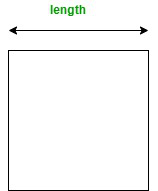• The perimeter of Square = 4a
• The area of Square = a2

Where ‘a’ is the length of the sides of a square

Rectangle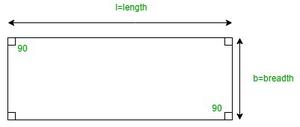• Perimeter of Rectangle = 2(l + b)
• The area of Rectangle = l × b

Where ‘l’ is Length and ‘b’ is Breadth

Triangle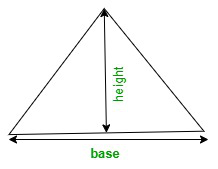• Area of Triangle = A = 1/2 × b × h

Where ‘b’ is the base of the triangle

and ‘h’ is the height of the triangle

Trapezoid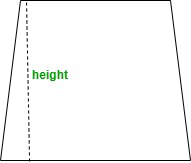• Area of Trapezoid = A =1/2 × (b1 + b2) × h

Where b1 and b2 are the bases of the Trapezoid

and, h is the height of the Trapezoid

Circle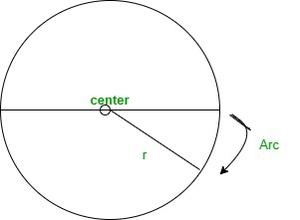• Area of Circle = A = π × r2
• Circumference of Circle = A = 2πr

Where ‘r’ is the radius of a Circle

Cube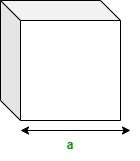• Surface Area of Cube = 6a2

Where ‘a’ is the length of the sides of the Cube

Cylinder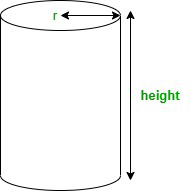• The curved surface area of Cylinder = 2πrh
• The total surface area of Cylinder = 2πr(r + h)
• The volume of Cylinder = V = πr2h

Where ‘r’ is the radius of the base of Cylinder

and ‘h’ is the height of Cylinder

Cone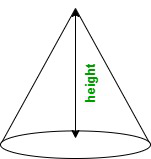• The curved surface area of a cone = πrl
• Total surface area of cone = πr(r + l) = πr[r + √(h2 + r2)]
• Volume of a Cone = V =1/2× πr2h

Here, ‘r’ is the radius of the base of Cone

and h is  height of the Cone

Sphere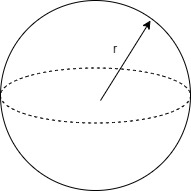• Surface Area of a Sphere = 4πr2
• The volume of a Sphere = 4/3 × πr3

Where r is the radius of the Sphere

### Sample Problems

Problem 1. If the radius of a circle is 14cm. Find the area of the given circle.

Solution:

Given

The radius of the circle is 14cm.

We have,

Area of the circle (A)=πr2

=>22/7 x 14 x 14

=>616cm2

Problem 2. Find the area of a triangle with a base of 12cm and a height of 8cm.

Solution:

Given

The base of the triangle is 12cm.

The height of the triangle is 8cm.

We have,

Area of triangle(A)=1/2 x b x h

=>1/2 x 12 x 8

=>48cm2

Problem 3. Find the perimeter of the given rectangle with a length of 10cm and breadth of 4cm.

Solution:

Given

The length of the rectangle is 10cm.

The breadth of the rectangle is 4cm.

We have,

Perimeter of rectangle(P)= 2(l+b)

=>2(10+4)

=>2 x 14

=>28cm

Problem 4. Find the perimeter of a square having a length of 5cm.

Solution:

Given

The length of the square is 5cm.

We have,

The perimeter of the square(P)= 4l

=> 4 x 5

=>20cm

Problem 5. Find the volume of a sphere having a radius of 9cm.

Solution:

Given

The radius of the sphere is 9cm.

We have

The volume of a sphere(V)=4/3 πr3

=>4/3 x 22/7 x (9)3

=>3054.85cm3

Problem 6. Calculate the area of a trapezoid having bases 8cm and 10cm and height of 12cm.

Solution:

Given

Let the bases of the trapezoid be b1 and b2 with values 8cm and 10cm respectively.

The height of the trapezoid is 12cm.

We have,

Area of Trapezoid = A =1/2 × (b1 + b2) × h

=>1/2 x (8 +10) x 12

=>1/2 x 18 x 12

=>216/2

=>108cm2

Problem 7. Find the volume of the given cone having a radius of 6cm and a height of 12cm.

Solution:

Given

The radius of the cone is 6cm.

The height of the given cone is 12cm

We have,

Volume of a Cone = V =1/2× πr2h

=>1/2 x 22/7 x (6)2 x 12

=>9504/14

=>678.85cm3

Problem 8. Calculate the curved surface area of a cylinder having a radius of 4cm and a height of 8cm.

Solution:

Given

The radius of the cylinder is 4cm.

The height of the cylinder is 8cm.

We have,

Curved surface area= 2πrh

=>2 x 22/7 x 4 x 8

=>201.14cm2

Problem 9. Calculate the area of a cube box having a side of 3cm.

Solution:

Given

The length of a side is 3cm.

We have,

Area of cube(A)= 6a2

=> 6 x 3 x3

=>54cm2

My Personal Notes arrow_drop_up
Related Tutorials